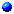Below are several examples of spreadsheets that I use both in the classroom and in presentations. No guidance is given about the mathematical topic covered by each one: You'll have to look elsewhere for that. These are here simply so people who attended a talk of mine on this subject can download what they saw. Just click on the title to download an Excel spreadsheet. These were made on my Macintosh in Excel 2001, so I have no idea if they will work on your PC. A version for Excel 95 is also available. Sorry if this doesn't work either.

###The Babylonian Method and Newton's Method

This spreadsheet enables you to use the classical "Babylonian method" and then Newton's method to find the square roots of real numbers. Visualization includes time series and the graphs of Newton's Method applied to x2 + c.

###The Logistic Population Model

This spreadsheet enables you to see the results of the iterative process known as the logistic population model both numerically and visually (as a time series and using graphical iteration). To understand this model, you should be familiar with the basic ideas concerning chaos (see, for example, the book Chaos.)

###Quadratic Iteration

This spreadsheet enables you to see the results of iterating the simple quadratic expression x2 + c both numerically and visually as a time series. Here c is a parameter that you can vary. In particular, you may witness chaotic behavior and the period doubling route to chaos using this tool. To understand what is going on, you should be familiar with the basic ideas concerning iteration and chaos (see, for example, the books Iteration and Chaos.)

###The Chaos Game

This spreadsheet contains a demo of the chaos game as well as an actual version of the game in which the die is rolled 10,000 times to produce the image. To use this spreadsheet, you need to understand what the chaos game is all about (see, for example, the book Fractals). Go to the Dynamical Systems and Technology Project to experiment with other versions of this game.

###Graphing Linear and Quadratic Equations

This spreadsheet allows the user to manipulate and animate graphs of linear and quadratic equations via scrollbars.